Biplots are two-dimensional plots that help to visualize relationships in high dimensional data. A previous article discusses how to interpret biplots for continuous variables. The biplot projects observations and variables onto the span of the first two principal components. The observations are plotted as markers; the variables are plotted as vectors. The observations and/or vectors are not usually on the same scale, so they need to be rescaled so that they fit on the same plot. There are four common scalings (GH, COV, JK, and SYM), which are discussed in the previous article.

This article shows how to create biplots in SAS. In particular, the goal is to create the biplots by using modern ODS statistical graphics. You can obtain biplots that use the traditional SAS/GRAPH system by using the %BIPLOT macro by Michael Friendly. The %BIPLOT macro is very powerful and flexible; it is discussed later in this article.

There are four ways to create biplots in SAS by using ODS statistical graphics:

• You can use PROC PRINQUAL in SAS/STAT software to create the COV biplot.
• If you have a license for SAS/GRAPH software (and SAS/IML software), you can use Friendly's %BIPLOT macro and use the OUT= option in the macro to save the coordinates of the markers and vectors. You can then use PROC SGPLOT to create a modern version of Friendly's biplot.
• You can use the matrix computations in SAS/IML to "manually" compute the coordinates of the markers and vectors. (These same computations are performed internally by the %BIPLOT macro.) You can use the Biplot module to create a biplot, or you can use the WriteBiplot module to create a SAS data set that contains the biplot coordinates. You can then use PROC SGPLOT to create the biplot.

For consistency with the previous article, all methods in this article standardize the input variables to have mean zero and unit variance (use the SCALE=STD option in the %BIPLOT macro). All biplots show projections of the same four-dimensional Fisher's iris data. The following DATA step assigns a blank label. If you do not supply an ID variable, some biplots display observations numbers.

```data iris; set Sashelp.iris; id = " "; /* create an empty label variable */ run;```

### Use PROC PRINQUAL to compute the COV biplot

The PRINQUAL procedure can perform a multidimensional preference analysis, which is visualized by using a MDPREF plot. The MDPREF plot is closely related to biplot (Jackson (1991), A User’s Guide to Principal Components, p. 204). You can get PROC PRINQUAL to produce a COV biplot by doing the following:

• Use the N=2 option to specify you want to compute two principal components.
• Use the MDPREF=1 option to specify that the procedure should not rescale the vectors in the biplot. By default, MDPREF=2.5 and the vectors appear 2.5 larger than they should be. (More on scaling vectors later.)
• Use the IDENTITY transformation so that the variables are not transformed in a nonlinear manner.

The following PROC PRINQUAL statements produce a COV biplot (click to enlarge):

```proc prinqual data=iris plots=(MDPref) n=2 /* project onto Prin1 and Prin2 */ mdpref=1; /* use COV scaling */ transform identity(SepalLength SepalWidth PetalLength PetalWidth); /* identity transform */ id ID; ods select MDPrefPlot; run;```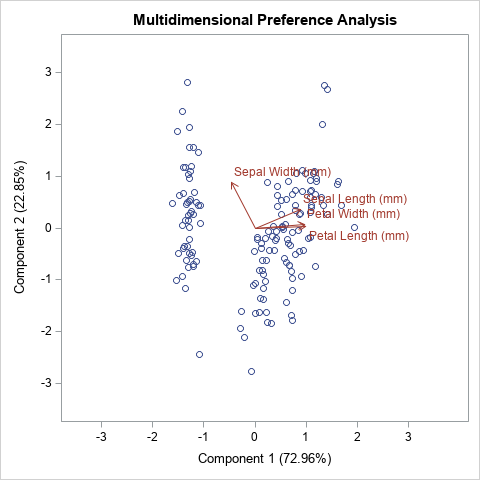### Use Friendly's %BIPLOT macro

Friendly's books [SAS System for Statistical Graphics (1991) and Visualizing Categorical Data (2000)] introduced many SAS data analysts to the power of using visualization to accompany statistical analysis, and especially the analysis of multivariate data. His macros use traditional SAS/GRAPH graphics from the 1990s. In the mid-2000s, SAS introduced ODS statistical graphics, which were released with SAS 9.2. Although the %BIPLOT macro does not use ODS statistical graphics directly, the macro supports the OUT= option, which enables you to create an output data set that contains all the coordinates for creating a biplot.

The following example assumes that you have downloaded the %BIPLOT macro and the %EQUATE macro from Michael Friendly's web site.

```/* A. Use %BIPLOT macro, which uses SAS/IML to compute the biplot coordinates. Use the OUT= option to get the coordinates for the markers and vectors. B. Transpose the data from long to wide form. C. Use PROC SGPLOT to create the biplot */ %let FACTYPE = SYM; /* options are GH, COV, JK, SYM */ title "Biplot: &FACTYPE, STD"; %biplot(data=iris, var=SepalLength SepalWidth PetalLength PetalWidth, id=id, factype=&FACTYPE, /* GH, COV, JK, SYM */ std=std, /* NONE, MEAN, STD */ scale=1, /* if you do not specify SCALE=1, vectors are auto-scaled */ out=biplotFriendly,/* write SAS data set with results */ symbols=circle dot, inc=1);   /* transpose from long to wide */ data Biplot; set biplotFriendly(where=(_TYPE_='OBS') rename=(dim1=Prin1 dim2=Prin2 _Name_=_ID_)) biplotFriendly(where=(_TYPE_='VAR') rename=(dim1=vx dim2=vy _Name_=_Variable_)); run;   proc sgplot data=Biplot aspect=1 noautolegend; refline 0 / axis=x; refline 0 / axis=y; scatter x=Prin1 y=Prin2 / datalabel=_ID_; vector x=vx y=vy / datalabel=_Variable_ lineattrs=GraphData2 datalabelattrs=GraphData2; xaxis grid offsetmin=0.1 offsetmax=0.2; yaxis grid; run;```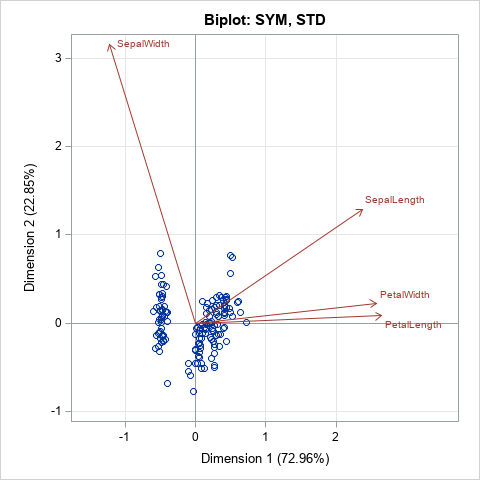Because you are using PROC SGPLOT to display the biplot, you can easily configure the graph. For example, I added grid lines, which are not part of the output from the %BIPLOT macro. You could easily change attributes such as the size of the fonts or add additional features such as an inset. With a little more work, you can merge the original data and the biplot data and color-code the markers by a grouping variable (such as Species) or by a continuous response variable.

Notice that the %BUPLOT macro supports a SCALE= option. The SCALE= option applies an additional linear scaling to the vectors. You can use this option to increase or decrease the lengths of the vectors in the biplot. For example, in the SYM biplot, shown above, the vectors are long relative to the range of the data. If you want to display vectors that are only 25% as long, you can specify SCALE=0.25. You can specify numbers greater than 1 to increase the vector lengths. For example, SCALE=2 will double the lengths of the vectors. If you omit the SCALE= option or set SCALE=0, then the %BIPLOT macro automatically scales the vectors to the range of the data. If you use the SCALE= option, you should tell the reader that you did so.

### SAS/IML modules that compute biplots

The %BIPLOT macro uses SAS/IML software to compute the locations of the markers and vectors for each type of biplot. I wrote three SAS/IML modules that perform the three steps of creating a biplot:

• The CalcBiplot module computes the projections of the observations and scores onto the first few principal components. This module (formerly named CalcPrinCompBiplot) was written in the mid-2000s and has been distributed as part of the SAS/IML Studio application. It returns the scores and vectors as SAS/IML matrices.
• The WriteBiplot module calls the CalcBiplot module and then writes the scores to a SAS data set called _SCORES and the vectors (loadings) to a SAS data set called _VECTORS. It also creates two macro variables, MinAxis and MaxAxis, which you can use if you want to equate the horizontal and vertical scales of the biplot axes.
• The Biplot function calls the WriteBiplot module and then calls PROC SGPLOT to create a biplot. It is the "raw SAS/IML" version of the %BIPLOT macro.

You can use the CalcBiplot module to compute the scores and vectors and return them in IML matrices. You can use the WriteBiplot module if you want that information in SAS data sets so that you can create your own custom biplot. You can use the Biplot module to create standard biplots. The Biplot and WriteBiplot modules are demonstrated in the next sections.

### Use the Biplot module in SAS/IML

The syntax of the Biplot module is similar to the %BIPLOT macro for most arguments. The input arguments are as follows:

• X: The numerical data matrix
• ID: A character vector of values used to label rows of X. If you pass in an empty matrix, observation numbers are used to label the markers. This argument is ignored if labelPoints=0.
• varNames: A character vector that contains the names of the columns of X.
• FacType: The type of biplot: 'GH', 'COV', 'JK', or 'SYM'.
• StdMethod: How the original variables are scaled: 'None', 'Mean', or 'Std'.
• Scale: A numerical scalar that specifies additional scaling applied to vectors. By default, SCALE=1, which means the vectors are not scaled. To shrink the vectors, specify a value less than 1. To lengthen the vectors, specify a value greater than 1. (Note: The %BIPLOT macro uses SCALE=0 as its default.)
• labelPoints: A binary 0/1 value. If 0 (the default) points are not labeled. If 1, points are labeled by the ID values. (Note: The %BIPLOT macro always labels points.)

The last two arguments are optional. You can specify them as keyword-value pairs outside of the parentheses. The following examples show how you can call the Biplot module in a SAS/IML program to create a biplot:

```ods graphics / width=480px height=480px; proc iml; /* assumes the modules have been previously stored */ load module=(CalcBiplot WriteBiplot Biplot); use sashelp.iris; read all var _NUM_ into X[rowname=Species colname=varNames]; close;   title "COV Biplot with Scaled Vectors and Labels"; run Biplot(X, Species, varNames, "COV", "Std") labelPoints=1; /* label obs */   title "JK Biplot: Relationships between Observations"; run Biplot(X, NULL, varNames, "JK", "Std");   title "JK Biplot: Automatic Scaling of Vectors"; run Biplot(X, NULL, varNames, "JK", "Std") scale=0; /* auto scale; empty ID var */   title "SYM Biplot: Vectors Scaled by 0.25"; run Biplot(X, NULL, varNames, "SYM", "Std") scale=0.25; /* scale vectors by 0.25 */```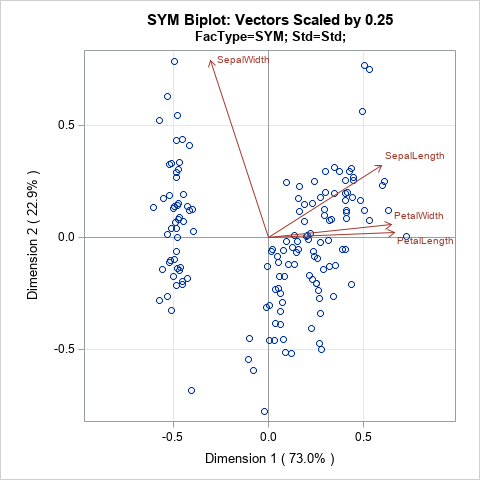The program creates four biplots, but only the last one is shown. The last plot uses the SCALE=0.25 option to rescale the vectors of the SYM biplot. You can compare this biplot to the SYM biplot in the previous section, which did not rescale the length of the vectors.

### Use the WriteBiplot module in SAS/IML

If you prefer to write an output data set and then create the biplot yourself, use the WriteBiplot module. After loading the modules and the data (see the previous section), you can write the biplot coordinates to the _Scores and _Vectors data sets, as follows. A simple DATA step appends the two data sets into a form that is easy to graph:

```run WriteBiplot(X, NULL, varNames, "JK", "Std") scale=0; /* auto scale vectors */ QUIT;   data Biplot; set _Scores _Vectors; /* append the two data sets created by the WriteBiplot module */ run;   title "JK Biplot: Automatic Scaling of Vectors"; title2 "FacType=JK; Std=Std"; proc sgplot data=Biplot aspect=1 noautolegend; refline 0 / axis=x; refline 0 / axis=y; scatter x=Prin1 y=Prin2 / ; vector x=vx y=vy / datalabel=_Variable_ lineattrs=GraphData2 datalabelattrs=GraphData2; xaxis grid offsetmin=0.1 offsetmax=0.1 min=&minAxis max=&maxAxis; yaxis grid min=&minAxis max=&maxAxis; run;```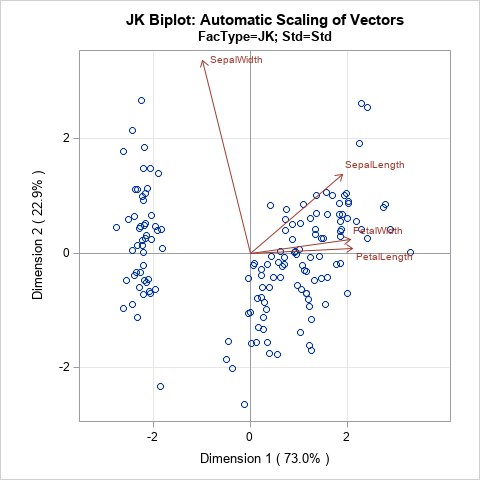In the program that accompanies this article, there is an additional example in which the biplot data is merged with the original data so that you can color-code the observations by using the Species variable.

### Summary

This article shows four ways to use modern ODS statistical graphics to create a biplot in SAS. You can create a COV biplot by using the PRINQUAL procedure. If you have a license for SAS/IML and SAS/GRAPH, you can use Friendly's %BIPLOT macro to write the biplot coordinates to a SAS data set, then use PROC SGPLOT to create the biplot. This article also presents SAS/IML modules that compute the same biplots as the %BIPLOT macro. The WriteBiplot module writes the data to two SAS data sets (_Score and _Vector), which can be appended and used to plot a biplot. This gives you complete control over the attributes of the biplot. Or, if you prefer, you can use the Biplot module in SAS/IML to automatically create biplots that are similar to Friendly's but are displayed by using ODS statistical graphics.

You can download the complete SAS program that is used in this article. For convenience, I have also created a separate file that defines the SAS/IML modules that create biplots.

The post Create biplots in SAS appeared first on The DO Loop.In grade school, students learn how to round numbers to the nearest integer. In later years, students learn variations, such as rounding up and rounding down by using the greatest integer function and least integer function, respectively. My sister, who is an engineer, learned a rounding method that rounds half-integers to the nearest even number. This method is called the round-to-even method. (Other names include the round-half-to-even method, the round-ties-to-even method, and "bankers' rounding.") When people first encounter the round-to-even method, they are often confused. Why would anyone use such a strange rounding scheme? This article describes the round-to-even method, explains why it is useful, and shows how to use SAS software to apply the round-to-even method.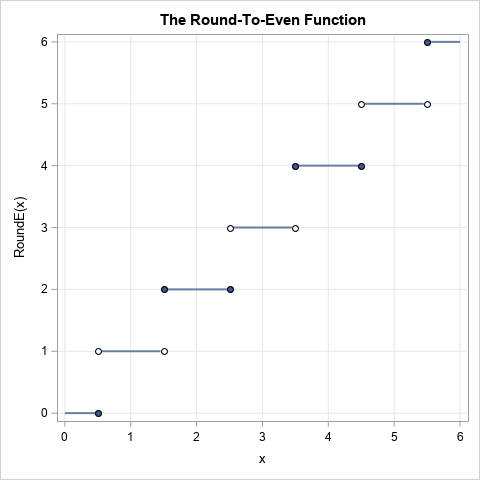### What is the round-to-even method of rounding?

The round-to-even method is used in engineering, finance, and computer science to reduce bias when you use rounded numbers to estimate sums and averages. The round-to-even method works like this:

• If the difference between the number and the nearest integer is less than 0.5, round to the nearest integer. This familiar rule is used by many rounding methods.
• If the difference between the number and the nearest integer is exactly 0.5, look at the integer part of the number. If the integer part is EVEN, round towards zero. If the integer part of the number is ODD, round away from zero. In either case, the rounded number is an even integer.

All rounding functions are discontinuous step functions that map the real numbers onto the integers. The graph of the round-to-even function is shown to the right.

I intentionally use the phrase "round away from zero" instead of "round up" because you can apply the rounding to positive or negative numbers. If you round the number -2.5 away from zero, you get -3. If you round the number -2.5 towards zero, you get -2. However, for simplicity, the remainder of the article uses positive numbers.

### Examples of the round-to-even method of rounding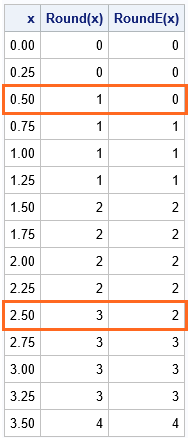For the number 2.5, which integer is closest to it? Well, it's a tie: 2.5 is a "half-integer" that is just as close to 2 as it is to 3. So which integer should you choose as THE rounded value? The traditional rounding method rounds the midpoint between two integers away from zero, so 2.5 is traditionally rounded to 3. This produces a systematic bias: all half-integers are rounded away from zero. This fact leads to biased estimates when you use the rounded data in an analysis.

To reduce this systematic bias, you can use the round-to-even method, which rounds some half-integers away from zero and others towards zero. For the round-to-even method, the number 2.5 rounds down to 2, whereas the number 3.5 rounds up to 4.

The table at the right shows some decimal values and the results of rounding the values under the standard method and the round-to-even method. The second column (Round(x)) shows the result of the traditional rounding method where all half-integers are rounded away from 0. The third column (RoundE(x)) is the round-to-even method that rounds half-integers to the nearest even integer. The red boxes indicate numbers for which the Round and RoundE functions produce different answers. Notice that for the round-to-even method, 50% of the half-integers round towards 0 and 50% round away from 0. In the table, 0.5 and 2.5 are rounded down by the round-to-even method, whereas 1.5 and 3.5 are rounded up.

### Why use the round-to-even method of rounding?

The main reason to use the round-to-even method is to avoid systematic bias when calculating with rounded numbers. One application involves mental arithmetic. If you want to estimate the sum (or mean) of a list of numbers, you can mentally round the numbers to the nearest integer and add up the numbers in your head. The round-to-even method helps to avoid bias in the estimate, especially if many of the values are half-integers.

Most computers use the round-to-even method for numerical computations. The round-to-even method has been a part of the IEEE standards for rounding since 1985.

### How to use the round-to-even method in SAS?

SAS software supports the ROUND function for standard rounding of numbers and the ROUNDE function ('E' for 'even') for round-to-even rounding. For example, the following DATA step produces the table that is shown earlier in this article:

```data Seq; keep x Round RoundEven; label Round = "Round(x)" RoundEven="RoundE(x)"; do x = 0 to 3.5 by 0.25; Round = round(x); /* traditional: half-integers rounded away from 0 */ RoundEven = rounde(x); /* round half-integers to the nearest even integer */ output; end; run;   proc print data=Seq noobs label; run;```

### An application: Estimate the average length of lengths with SAS

Although the previous sections discuss rounding values like 0.5 to the nearest integer, the same ideas apply when you round to the nearest tenth, hundredth, thousandth, etc. The next example rounds values to the nearest tenth. Values like 0.95, 1.05, 1.15, etc., are equidistant from the nearest tenth and can be rounded up or down, depending on the rounding method you choose. In SAS, you can use an optional argument to the ROUND and ROUNDE functions to specify the unit to which you want to round. For example, the expression ROUND(x, 0.1) rounds x to the nearest tenth.

An example in the SAS documentation for PROC UNIVARIATE contains the effective channel length (in microns) for 425 transistors from "Lot 1" of a production facility. In the data set, the lengths are recorded to two decimal places. What would be the impact on statistical measurements if the engineer had been lazy and decided to round the measurements to one decimal place, rather than typing all those extra digits?

The following SAS DATA step rounds the data to one decimal place (0.1 microns) by using the ROUND and ROUNDE functions. The call to PROC MEANS computes the mean and sum of the unrounded and rounded values. For the full-precision data, the estimate of the mean length is 1.011 microns. If you round the data by using the standard rounding method, the estimate shoots up to 1.018, which overestimates the average. In contrast, if you round the data by using the round-to-even method, the estimate is 1.014, which is closer to the average of the unrounded numbers (less biased). Similarly, the Sum column shows that the sum of the round-to-even values is much closer to the sum of the unrounded values.

```/* round real data to the nearest 0.1 unit */ data rounding; set Channel1; Round = round(Length, 0.1); /* traditional: round to nearest tenth */ RoundEven = rounde(Length, 0.1); /* use round-to-even method to round to nearest tenth */ /* create a binary indicator variable: Was x rounded up or down? */ RoundUp = (Round > Length); /* 1 if rounded up; 0 if rounded down */ RoundEvenUp = (RoundEven > Length); run;   proc means data=rounding sum mean ndec=3; label Length="True values" Round ="Rounded values" RoundEven="Round-to-even values"; var Length Round RoundEven; run;```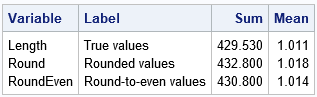As mentioned earlier, when you use the traditional rounding method, you introduce a bias every time you encounter a "half-unit" datum such as 0.95, 1.05, or 1.15. For this real data, you can count how many data were rounded up versus rounded down by each method. To get an unbiased result, you should round up the half-unit data about as often as you round down. The following call to PROC MEANS shows the proportion of data that are rounded up and rounded down by each method. The output shows that about 55% of the data are rounded up by the traditional rounding method, whereas a more equitable 50.1% of the values are rounded up by the round-to-even method.

```proc means data=rounding mean ndec=3; label RoundUp = "Proportion rounded up for ROUND" RoundEvenUp= "Proportion rounded up for ROUNDE"; var RoundUp RoundEvenUp; run;```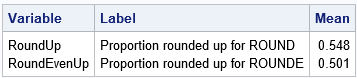This example illustrates a general truism: The round-to-even method is a less biased way to round data.

### Summary

This article explains the round-to-even method of rounding. This method is not universally taught, but it is taught to college students in certain disciplines. The method rounds most numbers to the nearest integer. However, half-integers, which are equally close to two integers, are rounded to the nearest EVEN integer, thus giving the method its name. This method reduces the bias when you use rounded values to estimate quantities such as sums, means, standard deviations, and so forth.

Whereas SAS provides separate ROUND and ROUNDE functions, other languages might default to the round-to-even method. For example, the round() function in python and the round function in R both implement the round-to-even method. Because some users are unfamiliar with this method of rounding, the R documentation provides an example and then explicitly states, "this is *good* behaviour -- do *NOT* report it as bug!"

The post Round to even appeared first on The DO Loop.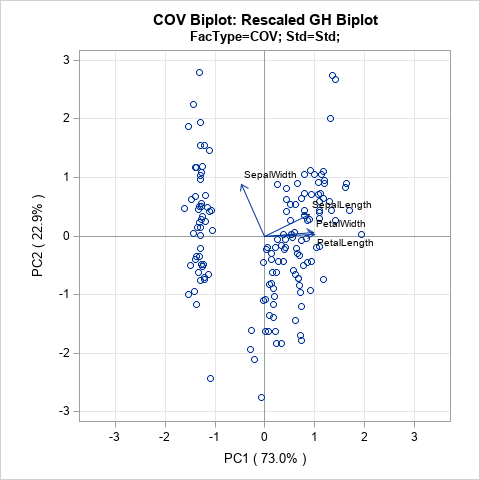Principal component analysis (PCA) is an important tool for understanding relationships in multivariate data. When the first two principal components (PCs) explain a significant portion of the variance in the data, you can visualize the data by projecting the observations onto the span of the first two PCs. In a PCA, this plot is known as a score plot. You can also project the variable vectors onto the span of the PCs, which is known as a loadings plot. See the article "How to interpret graphs in a principal component analysis" for a discussion of the score plot and the loadings plot.

A biplot overlays a score plot and a loadings plot in a single graph. An example is shown at the right. Points are the projected observations; vectors are the projected variables. If the data are well-approximated by the first two principal components, a biplot enables you to visualize high-dimensional data by using a two-dimensional graph.

In general, the score plot and the loadings plot will have different scales. Consequently, you need to rescale the vectors or observations (or both) when you overlay the score and loadings plots. There are four common choices of scaling. Each scaling emphasizes certain geometric relationships between pairs of observations (such as distances), between pairs of variables (such as angles), or between observations and variables. This article discusses the geometry behind two-dimensional biplots and shows how biplots enable you to understand relationships in multivariate data.

Some material in this blog post is based on documentation that I wrote in 2004 when I was working on the SAS/IML Studio product and writing the SAS/IML Studio User's Guide. The documentation is available online and includes references to the literature.

### The Fisher iris data

A previous article shows the score plot and loadings plot for a PCA of Fisher's iris data. For these data, the first two principal components explain 96% of the variance in the four-dimensional data. Therefore, these data are well-approximated by a two-dimensional set of principal components. For convenience, the score plot (scatter plot) and the loadings plot (vector plot) are shown below for the iris data. Notice that the loadings plot has a much smaller scale than the score plot. If you overlay these plots, the vectors would appear relatively small unless you rescale one or both plots.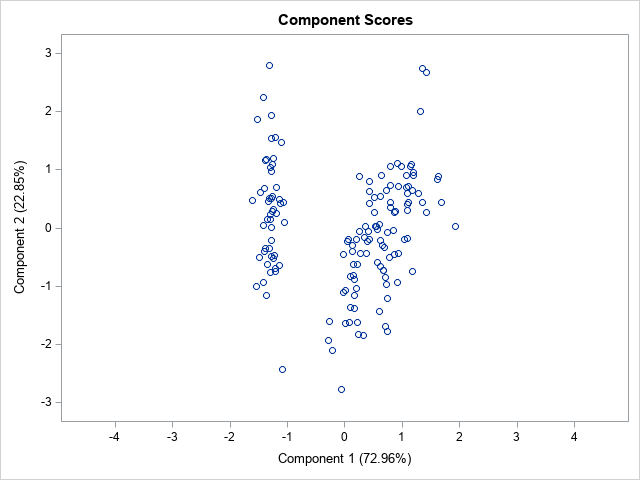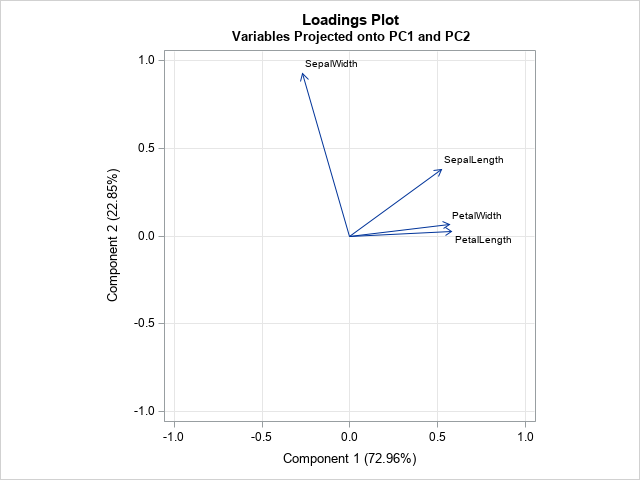### The mathematics of the biplot

You can perform a PCA by using a singular value decomposition of a data matrix that has N rows (observations) and p columns (variables). The first step in constructing a biplot is to center and (optionally) scale the data matrix. When variables are measured in different units and have different scales, it is usually helpful to standardize the data so that each column has zero mean and unit variance. The examples in this article use standardized data.

The heart of the biplot is the singular value decomposition (SVD). If X is the centered and scaled data matrix, then the SVD of X is
X = U L V`
where U is an N x N orthogonal matrix, L is a diagonal N x p matrix, and V is an orthogonal p x p matrix. It turns out that the principal components (PCs) of X`X are the columns of V and the PC scores are the columns of U. If the first two principal components explain most of the variance, you can choose to keep only the first two columns of U and V and the first 2 x 2 submatrix of L. This is the closest rank-two approximation to X. In a slight abuse of notation,
X ≈ U L V`
where now U, L, and V all have only two columns.

Since L is a diagonal matrix, you can write L = Lc L1-c for any number c in the interval [0, 1]. You can then write
X ≈ (U Lc)(L1-c V`)
= A B

This the factorization that is used to create a biplot. The most common choices for c are 0, 1, and 1/2.

### The four types of biplots

The choice of the scaling parameter, c, will linearly scale the observations and vectors separately. In addition, you can write X ≈ (β A) (B / β) for any constant β. Each choice for c corresponds to a type of biplot:

• When c=0, the vectors are represented faithfully. This corresponds to the GH biplot. If you also choose β = sqrt(N-1), you get the COV biplot.
• When c=1, the observations are represented faithfully. This corresponds to the JK biplot.
• When c=1/2, the observations and vectors are treated symmetrically. This corresponds to the SYM biplot.

### The GH biplot for variables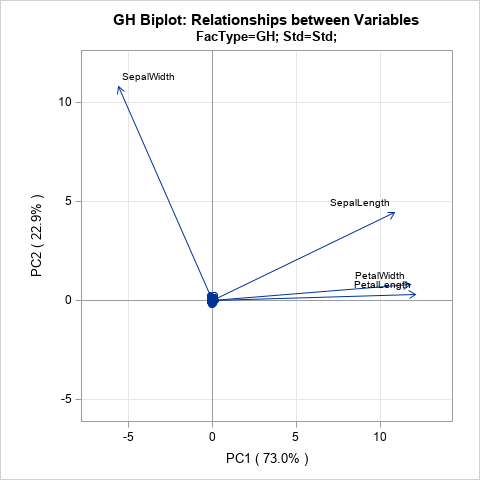If you choose c = 0, then A = U and B = L V`. The literature calls this biplot the GH biplot. I call it the "variable preserving" biplot because it provides the most faithful two-dimensional representation of the relationship between vectors. In particular:

• The length of each vector (a row of B) is proportional to the variance of the corresponding variable.
• The Euclidean distance between the i_th and j_th rows of A is proportional to the Mahalanobis distance between the i_th and j_th observations in the data.

In preserving the lengths of the vectors, this biplot distorts the Euclidean distance between points. However, the distortion is not arbitrary: it represents the Mahalanobis distance between points.

The GH biplot is shown to the right, but it is not very useful for these data. In choosing to preserve the variable relationships, the observations are projected onto a tiny region near the origin. The next section discusses an alternative scaling that is more useful for the iris data.

### The COV biplot

If you choose c = 0 and β = sqrt(N-1), then A = sqrt(N-1) U and B = L V` / sqrt(N-1). The literature calls this biplot the COV biplot. This biplot is shown at the top of this article. It has two useful properties:

• The length of each vector is equal to the variance of the corresponding variable.
• The Euclidean distance between the i_th and j_th rows of A is equal to the Mahalanobis distance between the i_th and j_th observations in the data.

In my opinion, the COV biplot is usually superior to the GH biplot.

### The JK biplot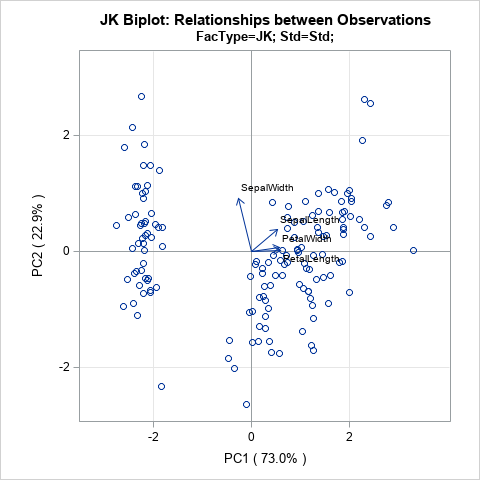If you choose c = 1, you get the JK biplot, which preserves the Euclidean distance between observations. Specifically, the Euclidean distance between the i_th and j_th rows of A is equal to the Euclidean distance between the i_th and j_th observations in the data.

In faithfully representing the observations, the angles between vectors are distorted by the scaling.

### The SYM biplot

If you choose c = 1/2, you get the SYM biplot (also called the SQ biplot), which attempts to treat observations and variables in a symmetric manner. Although neither the observations nor the vectors are faithfully represented, often neither representation is very distorted. Consequently, some people prefer the SYM biplot as a compromise between the COV and JK biplots. The SYM biplot is shown in the next section.

### How to interpret a biplot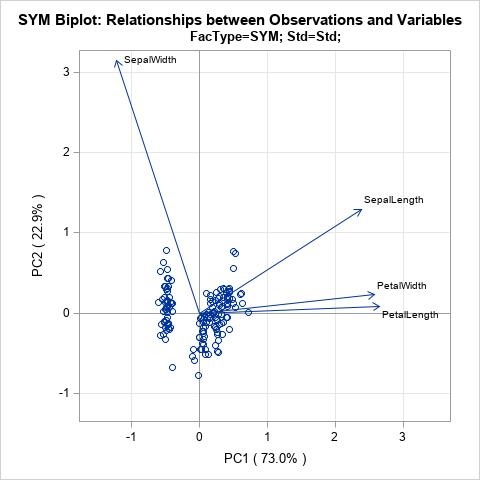As discussed in the SAS/IML Studio User's Guide, you can interpret a biplot in the following ways:

• The cosine of the angle between a vector and an axis indicates the importance of the contribution of the corresponding variable to the principal component.
• The cosine of the angle between pairs of vectors indicates correlation between the corresponding variables. Highly correlated variables point in similar directions; uncorrelated variables are nearly perpendicular to each other.
• Points that are close to each other in the biplot represent observations with similar values.
• You can approximate the relative coordinates of an observation by projecting the point onto the variable vectors within the biplot. However, you cannot use these biplots to estimate the exact coordinates because the vectors have been centered and scaled. You could extend the vectors to become lines and add tick marks, but that becomes messy if you have more than a few variables.

If you want to faithfully interpret the angles between vectors, you should equate the horizontal and vertical axes of the biplot, as I have done with the plots on this page.

If you apply these facts to the standardized iris data, you can make the following interpretations:

• The PetalLength and PetalWidth variables are the most important contributors to the first PC. The SepalWidth variable is the most important contributor to the second PC.
• The PetalLength and PetalWidth variables are highly correlated. The SepalWidth variable is almost uncorrelated with the other variables.
• Although I have suppressed labels on the points, you could label the points by an ID variable or by the observation number and use the relative locations to determine which flowers had measurements that were most similar to each other.

### Summary

This article presents an overview of biplots. A biplot is an overlay of a score plot and a loadings plot, which are two common plots in a principal component analysis. These two plots are on different scales, but you can rescale the two plots and overlay them on a single plot. Depending upon the choice of scaling, the biplot can provide faithful information about the relationship between variables (lengths and angles) or between observations (distances). It can also provide approximates relationships between variables and observations.

In a subsequent post, I will show how to use SAS to create the biplots in this article.

The post What are biplots? appeared first on The DO Loop.Understanding multivariate statistics requires mastery of high-dimensional geometry and concepts in linear algebra such as matrix factorizations, basis vectors, and linear subspaces. Graphs can help to summarize what a multivariate analysis is telling us about the data. This article looks at four graphs that are often part of a principal component analysis of multivariate data. The four plots are the scree plot, the profile plot, the score plot, and the pattern plot.

The graphs are shown for a principal component analysis of the 150 flowers in the Fisher iris data set. In SAS, you can create the graphs by using PROC PRINCOMP. By default, the scatter plots that display markers also label the markers by using an ID variable (such as name, state, patient ID, ...) or by using the observation number if you do not specify an ID variable. To suppress the labels, you can create an ID variable that contains a blank string, as follows:

```data iris; set Sashelp.iris; id = " "; /* create empty label variable */ run;```

The following statements create the four plots as part of a principal component analysis. The data are the measurements (in millimeters) of length and width of the sepal and petal for 150 iris flowers:

```ods graphics on; proc princomp data=iris /* use N= option to specify number of PCs */ STD /* optional: stdize PC scores to unit variance */ out=PCOut /* only needed to demonstate corr(PC, orig vars) */ plots=(scree profile pattern score); var SepalLength SepalWidth PetalLength PetalWidth; /* or use _NUMERIC_ */ ID id; /* use blank ID to avoid labeling by obs number */ ods output Eigenvectors=EV; /* to create loadings plot, output this table */ run;```

The principal components are linear combinations of the original data variables. Before we discuss the graph, let's identify the principal components and interpret their relationship to the original variables. The linear coefficients for the PCs (sometimes called the "loadings") are shown in the columns of the Eigenvectors table.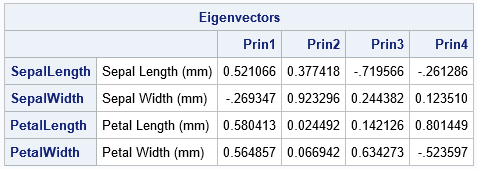• The first PC is the linear combination PC1 = 0.52*SepalLength – 0.27*SepalWidth + 0.58*PetalLength + 0.56*PetalWidth. You can interpret this as a contrast between the SepalWidth variable and an equally weighted sum of the other variables.
• For the second PC, the coefficients for the PetalLength and PetalWidth variables are very small. Therefore, the second PC is approximately PC2 ≈ 0.38*SepalLength + 0.92*SepalWidth. You can interpret this weighted sum as a vector that points mostly in the direction of the SepalWidth variable but has a small component in the direction of the SepalLength variable.
• In a similar way, the third PC is primarily a weighted contrast between the SepalLength and PetalWidth variables, with smaller contributions from the other variables.
• The fourth PC is a weighted contrast between the SepalWidth and PetalLength variables (with positive coefficients) and the SepalLength and PetalWidth variables (with negative coefficients).

Note that the principal components (which are based on eigenvectors of the correlation matrix) are not unique. If v is a PC vector, then so is -v. If you compare PCs from two different software packages, you might notice that a PC from one package is the negative of the same PC from another package.

### The scree plot

Recall that the main idea behind principal component analysis (PCA) is that most of the variance in high-dimensional data can be captured in a lower-dimensional subspace that is spanned by the first few principal components. You can therefore to "reduce the dimension" by choosing a small number of principal components to retain. But how many PCs should you retain? The scree plot is a line plot of the eigenvalues of the correlation matrix, ordered from largest to smallest. (If you use the COV option, it is a plot of the eigenvalues of the covariance matrix.)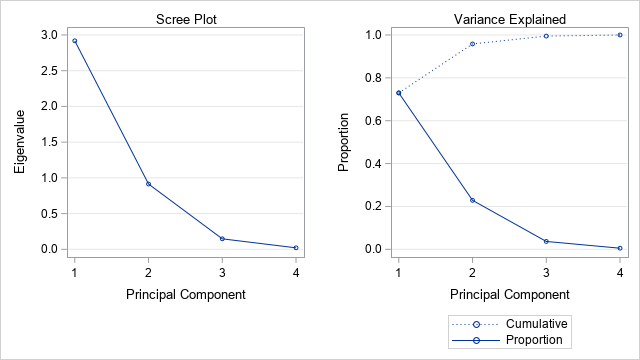You can use the scree plot as a graphical tool to help you choose how many PCs to retain. In the scree plot for the iris data, you can see (on the "Variance Explained" plot) that the first two eigenvalues explain about 96% of the variance in the four-dimensional data. This suggests that you should retain the first two PCs, and that a projection of the data onto the first to PCs will give you a good way to visualize the data in a low-dimensional linear subspace.

### The profile plot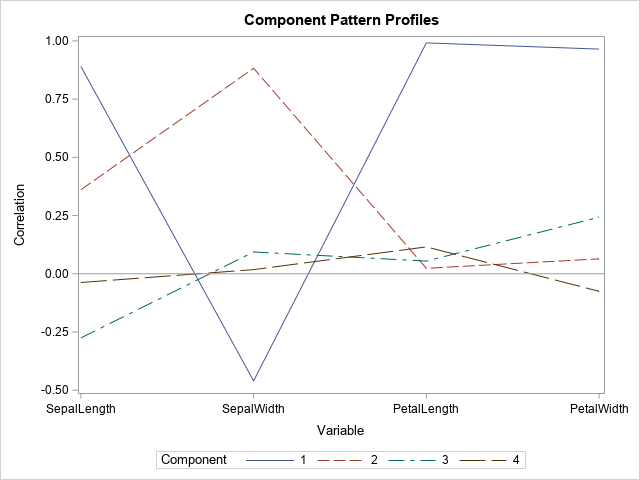The profile plot shows the correlations between each PC and the original variables. To some extent, you can guess the sign and the approximate magnitude of the correlations by looking at the coefficients that define each PC as a linear combination of the original variables. The correlations are shown in the following "Component Pattern Profiles" plot.

The profile plot reveals the following facts about the PCs:

• The first PC (solid blue line) is strongly positively correlated with SepalLength, PetalLength, and PetalWidth. It is moderately negatively correlated with SepalWidth.
• The second PC (dashed reddish line) is positively correlated with SepalLength and SepalWidth.
• The third and fourth PCs have only small correlations with the original variables.

The component pattern plot shows all pairwise correlations at a glance. However, if you are analyzing many variables (say 10 or more), the plot will become very crowded and hard to read. In that situation, the pattern plots are easier to read, as shown in the next section.

### The pattern plots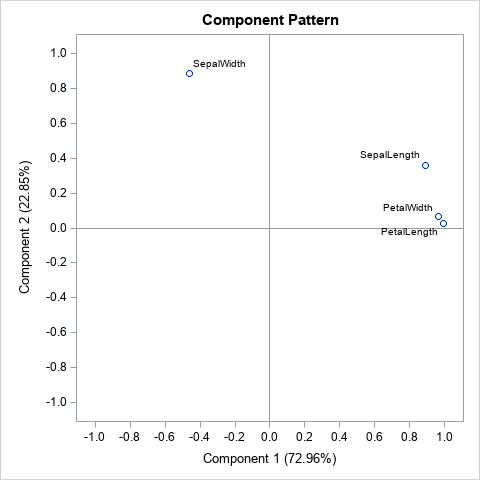The output from PROC PRINCOMP includes six "component pattern" plots, which show the correlations between the principal components and the original variables. Because there are four PCs, a component pattern plot is created for each pairwise combination of PCs: (PC1, PC2), (PC1, PC3), (PC1, PC4), (PC2, PC3), (PC2, PC4), and (PC3, PC4). In general, if there are k principal components, there are N(N-1)/2 pairwise combinations of PCs.

Each plot shows the correlations between the original variables and the PCs. For example, the graph shown to the right shows the correlations between the original variables and the first two PCs. Each point represents the correlations between an original variable and two PCs. The correlations with the first PC are plotted on the horizontal axis; the correlations with the second PC are plotted on the vertical axis.

Six graphs require a lot of space for what is essentially a grid of sixteen numbers. If you want to see the actual correlations in a table, you can call PROC CORR on the OUT= data set, as follows:

```/* what are the correlations between PCs and orig vars? */ proc corr data=PCOUT noprob nosimple; var SepalLength SepalWidth PetalLength PetalWidth; with Prin1-Prin4; run;```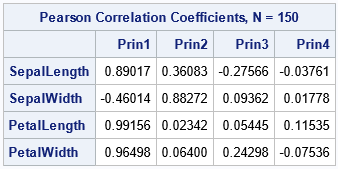If you know you want to keep only two PCs, you can use the N=2 option on the PROC PRINCOMP statement, which will reduce the number of graphs that are created.

### The score plotsThe score plots indicate the projection of the data onto the span of the principal components. As in the previous section, this four-dimensional example results in six score plots, one for each pairwise combination of PCs. You will get different plots if you create PCs for the covariance matrix (the COV option) as opposed to the correlation matrix (the default). Similarly, if you standardize the PCs (the STD option) or do not standardize them (the default), the corresponding score plot will be different.

The score plot for the first two PCs is shown. Notice that it uses equal scales for the axes. The graph shows that the first principal component separates the data into two clusters. The left cluster contains the flower from the Iris setosa species. You can see a few outliers, such as one setosa flower whose second PC score (about -2.5) is much smaller than the other setosa flowers.

ODS graphics provide an easy way to generate a quick look at the data. However, if you want to more control over the graphs, it is often simplest to output the results to a SAS data set and customize the plots by hand. You can use the OUT= option to write the PCs to a data set. The following call to PROC SGPLOT creates the same score plot but colors the markers by the Species variable and adds a grid of reference lines.

```title "Score Plot"; title2 "Observations Projected onto PC1 and PC2"; proc sgplot data=PCOut aspect=1; scatter x=Prin1 y=Prin2 / group=species; xaxis grid label="Component 1 (72.96%)"; yaxis grid label="Component 2 (22.85%)"; run;```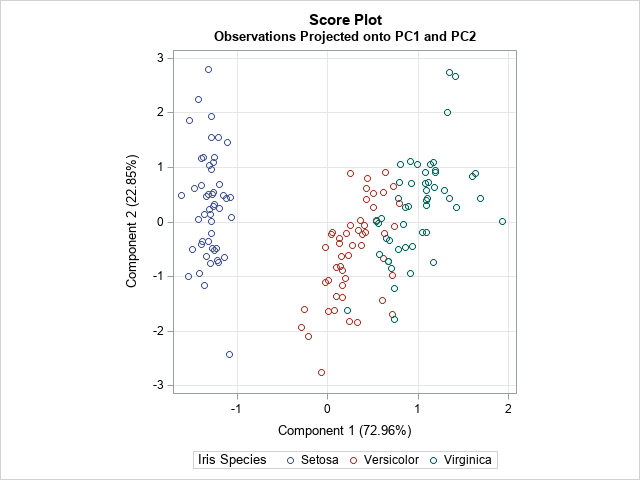A loadings plot is a plot of two columns of the Eigenvectors table. PROC PRINCOMP does not create a loadings plot automatically, but there are two ways to create it. One way is to use the ODS OUTPUT to write the Eigenvectors table to a SAS data set. The previous call to PROC PRINCOMP created a data set named EV. The following call to PROC SGPLOT creates a score plot that projects the observations onto the first two PCs.

```title "Loadings Plot"; title2 "Variables Projected onto PC1 and PC2"; proc sgplot data=EV aspect=1; vector x=Prin1 y=Prin2 / datalabel=Variable; xaxis grid label="Component 1 (72.96%)"; yaxis grid label="Component 2 (22.85%)"; run;```The loadings plot shows the relationship between the PCs and the original variables. You can use the graph to show how the original variables relate to the PCs, or the other way around. For example, the graph indicates that the PetalWidth and PetalLength variables point in the same direction as PC1. The graph also shows that the second PC is primarily in the direction of the SepalWidth variable, with a small shift towards the direction of the SepalLength variable. The second PC is essentially orthogonal to the and PetalWidth and PetalLength variables.

The second way to create a loadings plot is to use PROC FACTOR, as shown by the following statements. To documentation for PROC FACTOR compares the PROC FACTOR analysis to the PROC PRINCOMP analysis.

```proc factor data=iris N=2 /* use N= option to specify number of PCs */ method=principal plots=(initloadings(vector)); var SepalLength SepalWidth PetalLength PetalWidth; /* or use _NUMERIC_ */ run;```

### Summary

In summary, PROC PRINCOMP can compute a lot of graphs that are associated with a principal component analysis. This article shows how to interpret the most-used graphs. The scree plot is useful for determining the number of PCs to keep. The component pattern plot shows the correlations between the PCs and the original variables. The component pattern plots show similar information, but each plot displays the correlations between the original variables and a pair of PCs. The score plots project the observations onto a pair of PCs. The loadings plot project the original variables onto a pair of PCs.

When you analyze many variables, the number of graphs can be overwhelming. I suggest that you use the WHERE option in the ODS SELECT statement to restrict the number of pattern plots and score plots. For example, the following statement creates only two pattern plots and two score plots:

``` ods select Eigenvectors ScreePlot PatternProfilePlot where=(_label_ in: ('2 by 1','4 by 3')); /* limit pattern plots and score plots */```

There is one more plot that is sometimes used. It is called a "biplot" and it combines the information in a score plot and a loadings plot. I will discuss the biplot in a subsequent article.

The post How to interpret graphs in a principal component analysis appeared first on The DO Loop.Computing rates and proportions is a common task in data analysis. When you are computing several proportions, it is helpful to visualize how the rates vary among subgroups of the population. Examples of proportions that depend on subgroups include:

• Mortality rates for various types of cancers
• Incarceration rates by race

### Uncertainty in estimates

An important fact to remember is that the uncertainty in an estimate depends on the sample size. If a small college has 8 physics majors and 5 of them graduate in four years, the graduation rate in physics is 0.6. However, because of the small sample size, the uncertainty in that estimate is much greater than for a larger group, such as if the English department graduates 50 out of 80 students. Specifically, if the estimate of a binomial proportion is p, the standard error of the estimate is sqrt(p(1–p)/n), where n is the sample size. Thus for the physics students, the standard error is sqrt(0.6*0.4/8) = 0.17, whereas for the English majors, the standard error is sqrt(0.6*0.4/80) = 0.05.

Therefore, it is a good idea to incorporate some visual aspect of the uncertainty into any graph of proportions and rates. For analyses that involve dozens or hundreds of groups, you can use a funnel plot of proportions, which I have used to analyze adoption rates for children and immunization rates for kindergartens in North Carolina. When you have a smaller number of groups, a simple alternative is a dot plot with error bars that indicate either the standard error or a 95% confidence interval for the estimate. As I've explained, I prefer to display the confidence interval.

The Chronicle of Higher Education web site enables you to find the graduation rates for US colleges and universities. You can find the average graduation rate by states (50 groups) or by college (hundreds of groups). You can also find the graduation rate by race (five groups) for any individual college. Because most colleges have fewer Hispanic, Asian, and Native American students, it is important to indicate the sample size or the uncertainty in the empirical estimates.

I don't want to embarrass any small college, so the following data are fake but are typical of the group sizes that you might see in real data. Suppose a college has six majors, labeled as A, B, C, D, E, and F. The following SAS DATA step defines the number of students who graduated in four years (Grads) and the number of students in each cohort (Total).

```data Grads; input Major \$ Grads Total @@; datalines; A 10 22 B 10 32 C 17 25 D 4 7 E 8 14 F 16 28 ;```

### Manual computations of confidence intervals for proportions

If you use a simple Wald confidence interval, it is easy to write a short DATA step to compute the empirical proportions and a 95% confidence interval for each major:

```data GradRate; set Grads; GradRate = Grads / Total; p = Grads / Total; /* empirical proportion */ StdErr = sqrt(p*(1-p)/Total); /* standard error */ /* use Wald 95% CIs */ z = quantile("normal", 1-0.05/2); LCL = max(0, p - z*StdErr); /* LCL can't be less than 0 */ UCL = min(1, p + z*StdErr); /* UCL can't be less than 0 */ label p = "Proportion" LCL="Lower 95% CL" UCL="Upper 95% CL"; run;   proc print data=GradRate noobs label; var Major Grads Total p LCL UCL; run;```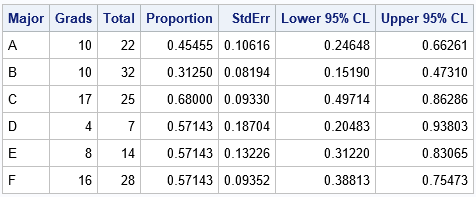The output shows that although majors D, E, and F have the same four-year graduation rate (57%), the estimate for the D group, which has only seven students, has twice as much variability as the estimate for the F group, which has four times as many students.

### Automating the computations by using PROC FREQ

Although it is easy enough to write a DATA step for the Wald CI, other types of confidence intervals are more complicated. The BINOMIAL option in the TABLES statement of PROC FREQ enables you to compute many different confidence intervals (CIs), including the Wald CI. In order to use these data in PROC FREQ, you need to convert the data from Event-Trials format to Event-Nonevent format. For each major, let Graduated="Yes" indicate the count of students who graduated in four years and let Graduated="No" indicate the count of the remaining students. The following data step converts the data and estimates the binomial proportion for each group:

```/* convert data to Event/Nonevent format */ data GradFreq; set Grads; Graduated = "Yes"; Count = Grads; output; Graduated = "No "; Count = Total-Grads; output; run;   /* Use PROC FREQ to analyze each group separately and compute the binomial CIs */ proc freq data=GradFreq noprint; by notsorted Major; tables Graduated / binomial(level='Yes' CL=wald); /* choose from among many confidence intervals */ weight Count; output out=FreqOut binomial; run;   proc print data=FreqOut noobs label; var Major N _BIN_ L_BIN U_BIN ; label _BIN_ = "Proportion" L_BIN="Lower 95% CL" U_BIN="Upper 95% CL"; run;```

The output is not shown because the estimates and CIs from PROC FREQ are identical to the estimates from the "manual" calculations in the previous section. However, by using PROC FREQ you can easily compute more sophisticated confidence intervals.

### Visualizing binomial proportions

As indicated earlier, it is useful to plot the proportions and confidence intervals. When you plot several proportions on the same graph, I recommend that you sort the data in some way, such as by the estimated proportions. If there are two groups that have the same proportion, you can use the size of the group to break the tie.

It can also be helpful to draw a reference line for the overall rate, regardless of group membership. (You can get the overall proportion by repeating the previous call to PROC FREQ but without using the BY statement.) For these data, the overall proportion of students who graduate in four years is 65/128 = 0.5078. Lastly, I think it is a good idea to add a table that shows the number of students in each major. You can use the YAXISTABLE statement to add that information to the graph, as follows:

```/* sort by estimated proportion; break ties by using CI */ proc sort data=FreqOut; by _BIN_ N; run;   title "Graduation Rates by College Major"; proc sgplot data=FreqOut; label _BIN_ = "Proportion" N="Number of Students"; scatter y=Major x=_BIN_ / xerrorlower=L_BIN xerrorupper=U_BIN; yaxistable N / y=Major location=inside position=left valueattrs=(size=9); refline 0.5078 / axis=x labelloc=inside label="Overall"; yaxis discreteorder=data offsetmax=0.1; /* preserve order of categories */ xaxis grid values=(0 to 1 by 0.1) valueshint; run;```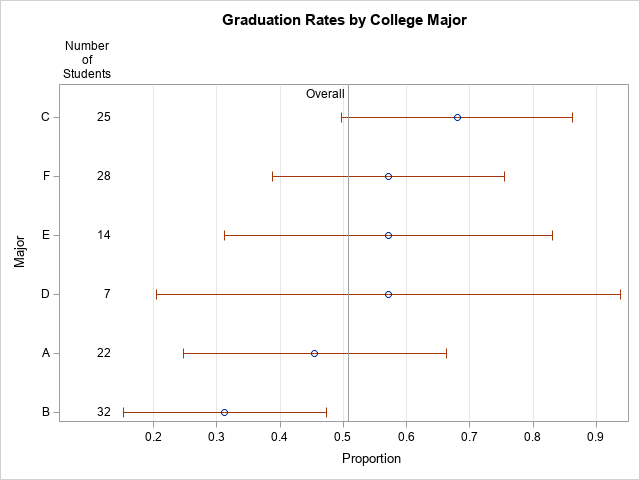The graph shows the range of graduation rates. The "error bars" are 95% CIs, which show that majors that have few students have larger uncertainty than majors that have more students. If there are 10 or more categories, I recommend that you use alternating color bands to make it easier for the reader to associate intervals with the majors.

In summary, this article shows how to use PROC FREQ to estimate proportions and confidence intervals for groups of binary data. A great way to convey the proportions to others is to graph the proportions and CIs. By including the sample size on the graph, readers can connect the uncertainty in the estimates to the sample size.

The post Compute and visualize binomial proportions in SAS appeared first on The DO Loop.Computing rates and proportions is a common task in data analysis. When you are computing several proportions, it is helpful to visualize how the rates vary among subgroups of the population. Examples of proportions that depend on subgroups include:

• Mortality rates for various types of cancers
• Incarceration rates by race

### Uncertainty in estimates

An important fact to remember is that the uncertainty in an estimate depends on the sample size. If a small college has 8 physics majors and 5 of them graduate in four years, the graduation rate in physics is 0.6. However, because of the small sample size, the uncertainty in that estimate is much greater than for a larger group, such as if the English department graduates 50 out of 80 students. Specifically, if the estimate of a binomial proportion is p, the standard error of the estimate is sqrt(p(1–p)/n), where n is the sample size. Thus for the physics students, the standard error is sqrt(0.6*0.4/8) = 0.17, whereas for the English majors, the standard error is sqrt(0.6*0.4/80) = 0.05.

Therefore, it is a good idea to incorporate some visual aspect of the uncertainty into any graph of proportions and rates. For analyses that involve dozens or hundreds of groups, you can use a funnel plot of proportions, which I have used to analyze adoption rates for children and immunization rates for kindergartens in North Carolina. When you have a smaller number of groups, a simple alternative is a dot plot with error bars that indicate either the standard error or a 95% confidence interval for the estimate. As I've explained, I prefer to display the confidence interval.

The Chronicle of Higher Education web site enables you to find the graduation rates for US colleges and universities. You can find the average graduation rate by states (50 groups) or by college (hundreds of groups). You can also find the graduation rate by race (five groups) for any individual college. Because most colleges have fewer Hispanic, Asian, and Native American students, it is important to indicate the sample size or the uncertainty in the empirical estimates.

I don't want to embarrass any small college, so the following data are fake but are typical of the group sizes that you might see in real data. Suppose a college has six majors, labeled as A, B, C, D, E, and F. The following SAS DATA step defines the number of students who graduated in four years (Grads) and the number of students in each cohort (Total).

```data Grads; input Major \$ Grads Total @@; datalines; A 10 22 B 10 32 C 17 25 D 4 7 E 8 14 F 16 28 ;```

### Manual computations of confidence intervals for proportions

If you use a simple Wald confidence interval, it is easy to write a short DATA step to compute the empirical proportions and a 95% confidence interval for each major:

```data GradRate; set Grads; GradRate = Grads / Total; p = Grads / Total; /* empirical proportion */ StdErr = sqrt(p*(1-p)/Total); /* standard error */ /* use Wald 95% CIs */ z = quantile("normal", 1-0.05/2); LCL = max(0, p - z*StdErr); /* LCL can't be less than 0 */ UCL = min(1, p + z*StdErr); /* UCL can't be less than 0 */ label p = "Proportion" LCL="Lower 95% CL" UCL="Upper 95% CL"; run;   proc print data=GradRate noobs label; var Major Grads Total p LCL UCL; run;```The output shows that although majors D, E, and F have the same four-year graduation rate (57%), the estimate for the D group, which has only seven students, has twice as much variability as the estimate for the F group, which has four times as many students.

### Automating the computations by using PROC FREQ

Although it is easy enough to write a DATA step for the Wald CI, other types of confidence intervals are more complicated. The BINOMIAL option in the TABLES statement of PROC FREQ enables you to compute many different confidence intervals (CIs), including the Wald CI. In order to use these data in PROC FREQ, you need to convert the data from Event-Trials format to Event-Nonevent format. For each major, let Graduated="Yes" indicate the count of students who graduated in four years and let Graduated="No" indicate the count of the remaining students. The following data step converts the data and estimates the binomial proportion for each group:

```/* convert data to Event/Nonevent format */ data GradFreq; set Grads; Graduated = "Yes"; Count = Grads; output; Graduated = "No "; Count = Total-Grads; output; run;   /* Use PROC FREQ to analyze each group separately and compute the binomial CIs */ proc freq data=GradFreq noprint; by notsorted Major; tables Graduated / binomial(level='Yes' CL=wald); /* choose from among many confidence intervals */ weight Count; output out=FreqOut binomial; run;   proc print data=FreqOut noobs label; var Major N _BIN_ L_BIN U_BIN ; label _BIN_ = "Proportion" L_BIN="Lower 95% CL" U_BIN="Upper 95% CL"; run;```

The output is not shown because the estimates and CIs from PROC FREQ are identical to the estimates from the "manual" calculations in the previous section. However, by using PROC FREQ you can easily compute more sophisticated confidence intervals.

### Visualizing binomial proportions

As indicated earlier, it is useful to plot the proportions and confidence intervals. When you plot several proportions on the same graph, I recommend that you sort the data in some way, such as by the estimated proportions. If there are two groups that have the same proportion, you can use the size of the group to break the tie.

It can also be helpful to draw a reference line for the overall rate, regardless of group membership. (You can get the overall proportion by repeating the previous call to PROC FREQ but without using the BY statement.) For these data, the overall proportion of students who graduate in four years is 65/128 = 0.5078. Lastly, I think it is a good idea to add a table that shows the number of students in each major. You can use the YAXISTABLE statement to add that information to the graph, as follows:

```/* sort by estimated proportion; break ties by using CI */ proc sort data=FreqOut; by _BIN_ N; run;   title "Graduation Rates by College Major"; proc sgplot data=FreqOut; label _BIN_ = "Proportion" N="Number of Students"; scatter y=Major x=_BIN_ / xerrorlower=L_BIN xerrorupper=U_BIN; yaxistable N / y=Major location=inside position=left valueattrs=(size=9); refline 0.5078 / axis=x labelloc=inside label="Overall"; yaxis discreteorder=data offsetmax=0.1; /* preserve order of categories */ xaxis grid values=(0 to 1 by 0.1) valueshint; run;```The graph shows the range of graduation rates. The "error bars" are 95% CIs, which show that majors that have few students have larger uncertainty than majors that have more students. If there are 10 or more categories, I recommend that you use alternating color bands to make it easier for the reader to associate intervals with the majors.

In summary, this article shows how to use PROC FREQ to estimate proportions and confidence intervals for groups of binary data. A great way to convey the proportions to others is to graph the proportions and CIs. By including the sample size on the graph, readers can connect the uncertainty in the estimates to the sample size.

The post Compute and visualize binomial proportions in SAS appeared first on The DO Loop.The EFFECT statement is supported by more than a dozen SAS/STAT regression procedures. Among other things, it enables you to generate spline effects that you can use to fit nonlinear relationships in data. Recently there was a discussion on the SAS Support Communities about how to interpret the parameter estimates of spline effects. This article answers that question by visualizing the spline effects.

### An overview of generated effects

Spline effects are powerful because they enable you to use parametric models to fit nonlinear relationships between an independent variable and a response. Using spline effects is not much different than use polynomial effects to fit nonlinear relationships. Suppose that a response variable, Y, appears to depend on an explanatory variable, X, in a complicated nonlinear fashion. If the relationship looks quadratic or cubic, you might try to capture the relationship by introducing polynomial effects. Instead of trying to model Y by X, you might try to use X, X2, and X3.

Strictly speaking, polynomial effects do not need to be centered at the origin. You could translate the polynomial by some amount, k, and use shifted polynomial effects such as (X-k), (X-k)2, and (X-k)3. Or you could combine these shifted polynomials with polynomials at the origin. Or use shifted polynomials that are shifted by different amounts, such as by the constants k1, k2, and k3.

Spline effects are similar to (shifted) polynomial effects. The constants (such as k1, k2, k3) that are used to shift the polynomials are called knots. Knots that are within the range of the data are called interior knots. Knots that are outside the range of the data are called exterior knots or boundary knots. You can read about the various kinds of spline effects that are supported by the EFFECT statement in SAS. Rather than rehash the mathematics, this article shows how you can use SAS to visualize a regression that uses splines. The visualization clarifies the meaning of the parameter estimates for the spline effects.

### Output and visualize spline effects

This section shows how to output the spline effects into a SAS data set and plot the spline effects. Suppose you want to predict the MPG_City variable (miles per gallon in the city) based on the engine size. Because we will be plotting curves, the following statements sort the data by the EngineSize variable. Then the OUTDESIGN= option on the PROC GLMSELECT statement writes the spline effects to the Splines data set. For this example, I am using restricted cubic splines and four evenly spaced internal knots, but the same ideas apply to any choice of spline effects.

```/* Fit data by using restricted cubic splines. The EFFECT statement is supported by many procedures: GLIMMIX, GLMSELECT, LOGISTIC, PHREG, ... */ title "Restricted TPF Splines"; title2 "Four Internal Knots"; proc glmselect data=cars outdesign(addinputvars fullmodel)=Splines; /* data set contains spline effects */ effect spl = spline(EngineSize / details /* define spline effects */ naturalcubic basis=tpf(noint) /* natural cubic splines, omit constant effect */ knotmethod=equal(4)); /* 4 evenly spaced interior knots */ model mpg_city = spl / selection=none; /* fit model by using spline effects */ ods select ParameterEstimates SplineKnots; ods output ParameterEstimates=PE; quit;```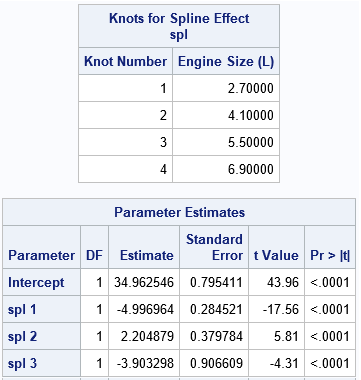The SplineKnots table shows the locations of the internal knots. There are four equally spaced knots because the procedure used the KNOTMETHOD=EQUAL(4) option. The ParameterEstimates table shows estimates for the regression coefficients for the spline effects, which are named "Spl 1", "Spl 2", and so forth. In the Splines data set, the corresponding variables are named Spl_1, Spl_2, and so forth.

But what do these spline effects look like? The following statements plot the spline effects versus the EngineSize variable, which is the variable from which the effects are generated:

```proc sgplot data=Splines; series x=EngineSize y=Intercept / curvelabel; series x=EngineSize y=spl_1 / curvelabel; series x=EngineSize y=spl_2 / curvelabel; series x=EngineSize y=spl_3 / curvelabel; refline 2.7 4.1 5.5 / axis=x lineattrs=(color="lightgray"); refline 6.9 / axis=x label="upper knot" labelloc=inside lineattrs=(color="lightgray"); yaxis label="Spline Effect"; run;```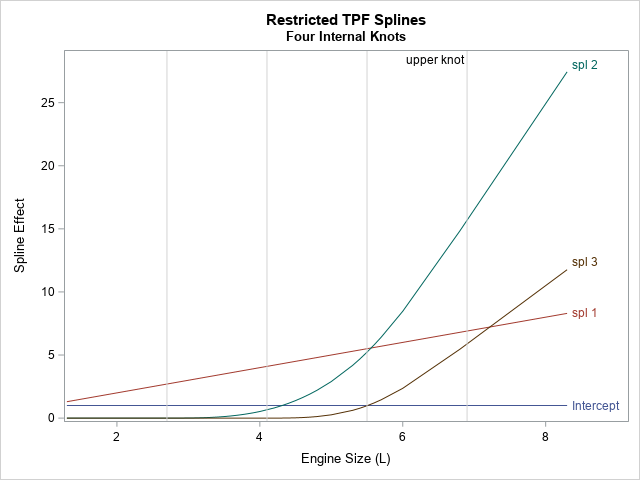As stated in the documentation for the NATURALCUBIC option, these spline effects include "an intercept, the polynomial X, and n – 2 functions that are all linear beyond the largest knot," where n is the number of knots. This example uses n=4 knots, so Spl_2 and Spl_3 are the cubic splines. You will also see different spline effects if you change to one of the other supported spline methods, such as B-splines or the truncated power functions. Try it!

The graph shows that the natural cubic splines are reminiscent of polynomial effects, but there are a few differences:

• The spline effects (spl_2 and spl_3) are shifted away from the origin. The spl_2 effect is shifted by 2.7 units, which is the location of the first internal knot. The spl_3 effect is shifted by 4.1 units, which is the location of the second internal knot.
• The spline effects are 0 when EngineSize is less than the first knot position (2.7). Not all splines look like this, but these effects are based on truncated power functions (the TPF option).
• The spline effects are linear when EngineSize is greater than the last knot position (6.9). Not all splines look like this, but these effects are restricted splines.

### Predicted values are linear combinations of the spline effects

Visualizing the shapes of the spline effects enable you to make sense of the ParameterEstimates table. As in all linear regression, the predicted value is a linear combination of the design variables. In this case, the predicted values are formed by
Pred = 34.96 – 5*Spl_1 + 2.2*Spl_2 – 3.9*Spl_3
You can use the SAS DATA set or PROC IML to compute that linear combination of the spline effects. The following graph shows the predicted curve and overlays the locations of the knots: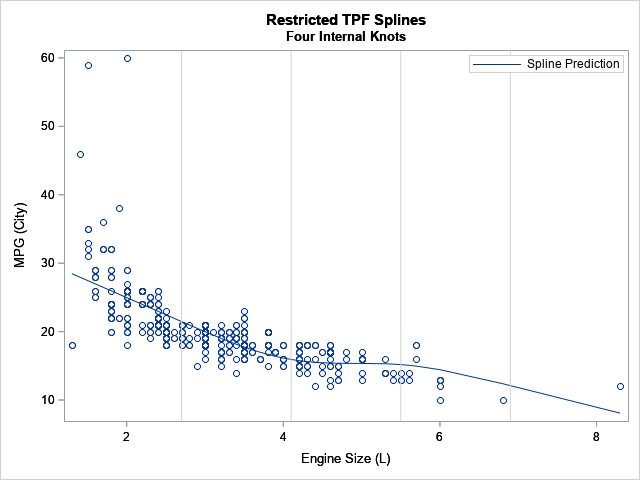The coefficient for Spl_1 is the largest effect (after the intercept). In the graph, you can see the general trend has an approximate slope of -5. The coefficient for the Spl_2 effect is 2.2, and you can see that the predicted values change slope between the second and third knots due to adding the Spl_2 effect. Without the Spl_3 effect, the predicted values would continue to rise after the third knot, but by adding in a negative multiple of Spl_3, the predicted values turn down again after the third knot.

Notice that the prediction function for the restricted cubic spline regression is linear before the first knot and after the last knot. The prediction function models nonlinear relationships between the interior knots.

### Summary

In summary, the EFFECT statement in SAS regression procedures can generate spline effects for a continuous explanatory variable. The EFFECT statement supports many different types of splines. This article gives an example of using natural cubic splines (also called restricted cubic splines), which are based on the truncated power function (TPF) splines of degree 3. By outputting the spline effects to a data set and graphing them, you can get a better understanding of the meaning of the estimates of the regression coefficients. The predicted values are a linear combination of the spline effects, so the magnitude and sign of the regression coefficients indicate how the spline effects combine to predict the response.

The post Visualize a regression with splines appeared first on The DO Loop.I recently wrote about how to use PROC TTEST in SAS/STAT software to compute the geometric mean and related statistics. This prompted a SAS programmer to ask a related question. Suppose you have dozens (or hundreds) of variables and you want to compute the geometric mean of each. What is the best way to obtain these geometric means?

As I mentioned in the previous post, the SAS/IML language supports the GEOMEAN function, so you compute the geometric means by iterating over each column in a data matrix. If you do not have SAS/IML software, you can use PROC UNIVARIATE in Base SAS. The UNIVARIATE procedure supports the OUTTABLE= option, which creates a SAS data set that contains many univariate statistics, including the geometric mean.

For example, suppose you want to compute the geometric means for all numeric variables in the Sashelp.Cars data set. You can use the OUTTABLE= option to write the output statistics to a data set and then print only the column that contains the geometric mean, as follows:

```proc univariate data=Sashelp.Cars outtable=DescStats noprint; var _NUMERIC_; run;   proc print data=DescStats noobs; var _var_ _GEOMEAN_; run;```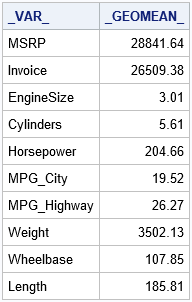This method also works if your data contain a classification variable and you want to compute the geometric mean for each level of the classification variable. For example, the following statements compute the geometric means for two variables for each level of the Origin variable, which has the values "Asia", "Europe", and "USA":

```proc univariate data=Sashelp.Cars outtable=DescStatsClass noprint; class Origin; var MPG_City Horsepower; run;   proc print data=DescStatsClass noobs; var _var_ Origin _GEOMEAN_; run;```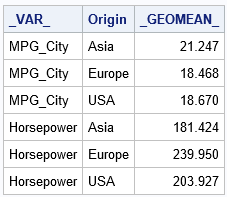In summary, if you want to use Base SAS to compute the geometric mean (or any of almost 50 other descriptive statistics) for many variables, use the OUTTABLE= option of PROC UNIVARIATE.

The post Compute the geometric mean for many variables in SAS appeared first on The DO Loop.In a previous article, I mentioned that the VLINE statement in PROC SGPLOT is an easy way to graph the mean response at a set of discrete time points. I mentioned that you can choose three options for the length of the "error bars": the standard deviation of the data, the standard error of the mean, or a confidence interval for the mean. This article explains and compares these three options. Which one you choose depends on what information you want to convey to your audience. As I will show, some of the statistics are easier to interpret than others. At the end of this article, I tell you which statistic I recommend.

### Sample data

The following DATA step simulates data at four time points. The data at each time point are normally distributed, but the mean, standard deviation, and sample size of the data vary for each time point.

```data Sim; label t = "Time"; array mu _temporary_ (80 78 78 79); /* mean */ array sigma _temporary_ ( 1 2 2 3); /* std dev */ array N _temporary_ (36 32 28 25); /* sample size */ call streaminit(12345); do t = 1 to dim(mu); do i = 1 to N[t]; y = rand("Normal", mu[t], sigma[t]); /* Y ~ N(mu[i], sigma[i]) */ output; end; end; run;   title "Response by Time"; ods graphics / width=400px height=250px; proc sgplot data=Sim; vbox y / category=t connect=mean; run;```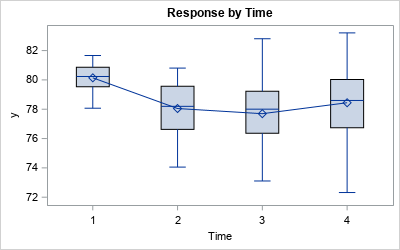The box plot shows the schematic distribution of the data at each time point. The boxes use the interquartile range and whiskers to indicate the spread of the data. A line connects the means of the responses at each time point.

A box plot might not be appropriate if your audience is not statistically savvy. A simpler display is a plot of the mean for each time point and error bars that indicate the variation in the data. But what statistic should you use for the heights of the error bars? What is the best way to show the variation in the response variable?

### Relationships between sample standard deviation, SEM, and CLM

Before I show how to plot and interpret the various error bars, I want to review the relationships between the sample standard deviation, the standard error of the mean (SEM), and the (half) width of the confidence interval for the mean (CLM). These statistics are all based on the sample standard deviation (SD). The SEM and width of the CLM are multiples of the standard deviation, where the multiplier depends on the sample size:

• The SEM equals SD / sqrt(N). That is, the standard error of the mean is the standard deviation divided by the square root of the sample size.
• The width of CLM is a multiple of the SEM. For large samples, the multiple for a 95% confidence interval is approximately 1.96. In general, suppose the significance level is α and you are interested in 100(1-α)% confidence limits. Then the multiplier is a quantile of the t distribution with N-1 degrees of freedom, often denoted by t*1-α/2, N-1.

You can use PROC MEANS and a short DATA step to display the relevant statistics that show how these three statistics are related:

```/* Optional: Compute SD, SEM, and half-width of CLM (not needed for plotting) */ proc means data=Sim noprint; class t; var y; output out=MeanOut N=N stderr=SEM stddev=SD lclm=LCLM uclm=UCLM; run;   data Summary; set MeanOut(where=(t^=.)); CLMWidth = (UCLM-LCLM)/2; /* half-width of CLM interval */ run;   proc print data=Summary noobs label; format SD SEM CLMWidth 6.3; var T SD N SEM CLMWidth; run;```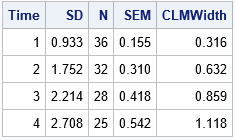The table shows the standard deviation (SD) and the sample size (N) for each time point. The SEM column is equal to SD / sqrt(N). The CLMWidth value is a little more than twice the SEM value. (The multiplier depends on N; For these data, it ranges from 2.03 to 2.06.)

As shown in the next section, the values in the SD, SEM, and CLMWidth columns are the lengths of the error bars when you use the STDDEV, STDERR, and CLM options (respectively) to the LIMITSTAT= option on the VLINE statement in PROC SGPLOT.

### Visualize and interpret the choices of error bars

Let's plot all three options for the error bars on the same scale, then discuss how to interpret each graph. Several interpretations use the 68-95-99.7 rule for normally distributed data. The following statements create the three line plots with error bars:

```%macro PlotMeanAndVariation(limitstat=, label=); title "VLINE Statement: LIMITSTAT = &limitstat"; proc sgplot data=Sim noautolegend; vline t / response=y stat=mean limitstat=&limitstat markers; yaxis label="&label" values=(75 to 82) grid; run; %mend;   title "Mean Response by Time Point"; %PlotMeanAndVariation(limitstat=STDDEV, label=Mean +/- Std Dev); %PlotMeanAndVariation(limitstat=STDERR, label=Mean +/- SEM); %PlotMeanAndVariation(limitstat=CLM, label=Mean and CLM);```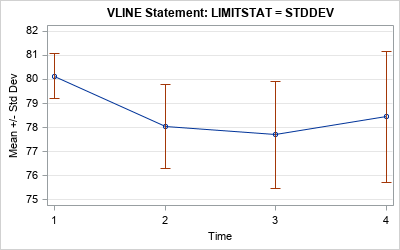#### Use the standard deviations for the error bars

In the first graph, the length of the error bars is the standard deviation at each time point. This is the easiest graph to explain because the standard deviation is directly related to the data. The standard deviation is a measure of the variation in the data. If the data at each time point are normally distributed, then (1) about 64% of the data have values within the extent of the error bars, and (2) almost all the data lie within three times the extent of the error bars.

The main advantage of this graph is that a "standard deviation" is a term that is familiar to a lay audience. The disadvantage is that the graph does not display the accuracy of the mean computation. For that, you need one of the other statistics.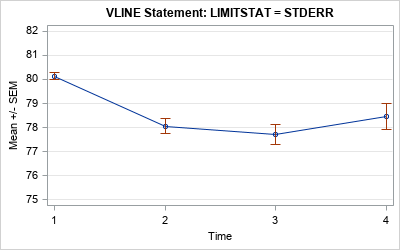#### Use the standard error for the error bars

In the second graph, the length of the error bars is the standard error of the mean (SEM). This is harder to explain to a lay audience because it in an inferential statistic. A qualitative explanation is that the SEM shows the accuracy of the mean computation. Small SEMs imply better accuracy than larger SEMs.

A quantitative explanation requires using advanced concepts such as "the sampling distribution of the statistic" and "repeating the experiment many times." For the record, the SEM is an estimate of the standard deviation of the sampling distribution of the mean. Recall that the sampling distribution of the mean can be understood in terms of repeatedly drawing random samples from the population and computing the mean for each sample. The standard error is defined as the standard deviation of the distribution of the sample means.

The exact meaning of the SEM might be difficult to explain to a lay audience, but the qualitative explanation is often sufficient.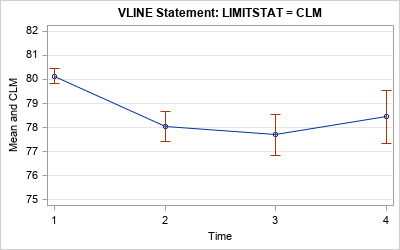#### Use a confidence interval of the mean for the error bars

In the third graph, the length of the error bars is a 95% confidence interval for the mean. This graph also displays the accuracy of the mean, but these intervals are about twice as long as the intervals for the SEM.

The confidence interval for the mean is hard to explain to a lay audience. Many people incorrectly think that "there is a 95% chance that the population mean is in this interval." That statement is wrong: either the population mean is in the interval or it isn't. There is no probability involved! The words "95% confidence" refer to repeating the experiment many times on random samples and computing a confidence interval for each sample. The true population mean will be in about 95% of the confidence intervals.

### Conclusions

In summary, there are three common statistics that are used to overlay error bars on a line plot of the mean: the standard deviation of the data, the standard error of the mean, and a 95% confidence interval for the mean. The error bars convey the variation in the data and the accuracy of the mean estimate. Which one you use depends on the sophistication of your audience and the message that you are trying to convey.

My recommendation? Despite the fact that confidence intervals can be misinterpreted, I think that the CLM is the best choice for the size of the error bars (the third graph). If I am presenting to a statistical audience, the audience understands the CLMs. For a less sophisticated audience, I do not dwell on the probabilistic interpretation of the CLM but merely say that the error bars "indicate the accuracy of the mean."

As explained previously, each choice has advantages and disadvantages. What choice do you make and why? You can share your thoughts by leaving a comment.

The post What statistic should you use to display error bars for a mean? appeared first on The DO Loop.I frequently see questions on SAS discussion forums about how to compute the geometric mean and related quantities in SAS. Unfortunately, the answers to these questions are sometimes confusing or even wrong. In addition, some published papers and web sites that claim to show how to calculate the geometric mean in SAS contain wrong or misleading information.

This article shows how to compute the geometric mean, the geometric standard deviation, and the geometric coefficient of variation in SAS. It first shows how to use PROC TTEST to compute the geometric mean and the geometric coefficient of variation. It then shows how to compute several geometric statistics in the SAS/IML language.

For an introduction to the geometric mean, see "What is a geometric mean." For information about the (arithmetic) coefficient of variation (CV) and its applications, see the article "What is the coefficient of variation?"

### Compute the geometric mean and geometric CV in SAS

As discussed in my previous article, the geometric mean arises naturally when positive numbers are being multiplied and you want to find the average multiplier. Although the geometric mean can be used to estimate the "center" of any set of positive numbers, it is frequently used to estimate average values in a set of ratios or to compute an average growth rate.

The TTEST procedure is the easiest way to compute the geometric mean (GM) and geometric CV (GCV) of positive data. To demonstrate this, the following DATA step simulates 100 random observations from a lognormal distribution. PROC SGPLOT shows a histogram of the data and overlays a vertical line at the location of the geometric mean.

```%let N = 100; data Have; call streaminit(12345); do i = 1 to &N; x = round( rand("LogNormal", 3, 0.8), 0.1); /* generate positive values */ output; end; run;   title "Geometric Mean of Skewed Positive Data"; proc sgplot data=Have; histogram x / binwidth=10 binstart=5 showbins; refline 20.2 / axis=x label="Geometric/Mean" splitchar="/" labelloc=inside lineattrs=GraphData2(thickness=3); xaxis values=(0 to 140 by 10); yaxis offsetmax=0.1; run;```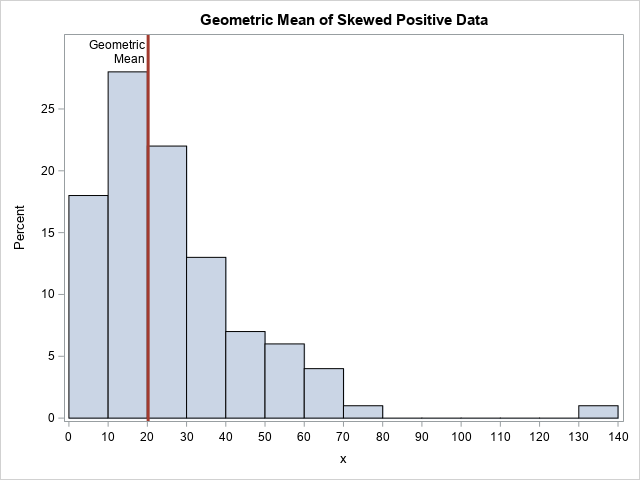Where is the "center" of these data? That depends on your definition. The mode of this skewed distribution is close to x=15, but the arithmetic mean is about 26.4. The mean is pulled upwards by the long right tail. It is a mathematical fact that the geometric mean of data is always less than the arithmetic mean. For these data, the geometric mean is 20.2.

To compute the geometric mean and geometric CV, you can use the DIST=LOGNORMAL option on the PROC TTEST statement, as follows:

```proc ttest data=Have dist=lognormal; var x; ods select ConfLimits; run;```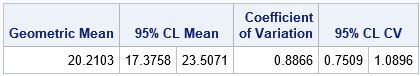The geometric mean, which is 20.2 for these data, estimates the "center" of the data. Notice that the procedure does not report the geometric standard deviation (or variance), but instead reports the geometric coefficient of variation (GCV), which has the value 0.887 for this example. The documentation for the TTEST procedure explains why the GCV is the better measure of variation: "For lognormal data, the CV is the natural measure of variability (rather than the standard deviation) because the CV is invariant to multiplication of [the data]by a constant."

You might wonder whether data need to be lognormally distributed to use this table. The answer is that the data do not need to be lognormally distributed to use the geometric mean and geometric CV. However, the 95% confidence intervals for these quantities assume log-normality.

### Definitions of geometric statistics

As T. Kirkwood points out in a letter to the editors of Biometric (Kirkwood, 1979), if data are lognormally distributed as LN(μ σ), then

• The quantity GM = exp(μ) is the geometric mean. It is estimated from a sample by the quantity exp(m), where m is the arithmetic mean of the log-transformed data.
• The quantity GSD = exp(σ) is defined to be the geometric standard deviation. The sample estimate is exp(s), where s is the standard deviation of the log-transformed data.
• The geometric standard error (GSE) is defined by exponentiating the standard error of the mean of the log-transformed data. Geometric confidence intervals are handled similarly.
• Kirkwood's proposal for the geometric coefficient of variation (GCV) is not generally used. Instead, the accepted definition of the GCV is GCV = sqrt(exp(σ2) – 1), which is the definition that is used in SAS. The estimate for the GCV is sqrt(exp(s2) – 1).

You can use these formulas to compute the geometric statistics for any positive data. However, only for lognormal data do the statistics have a solid theoretical basis: transform to normality, compute a statistic, apply the inverse transform.

### Compute the geometric mean in SAS/IML

You can use the SAS/IML language to compute the geometric mean and other "geometric statistics" such as the geometric standard deviation and the geometric CV. The GEOMEAN function is a built-in SAS/IML function, but the other statistics are implemented by explicitly computing statistics of the log-transformed data, as described in the previous section:

```proc iml; use Have; read all var "x"; close; /* read in positive data */ GM = geomean(x); /* built-in GEOMEAN function */ print GM;   /* To estimate the geometric mean and geometric StdDev, compute arithmetic estimates of log(X), then EXP transform the results. */ n = nrow(x); z = log(x); /* log-transformed data */ m = mean(z); /* arithmetic mean of log(X) */ s = std(z); /* arithmetic std dev of log(X) */ GM2 = exp(m); /* same answer as GEOMEAN function */ GSD = exp(s); /* geometric std dev */ GCV = sqrt(exp(s**2) - 1); /* geometric CV */ print GM2 GSD GCV;```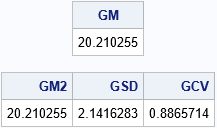Note that the GM and GCV match the output from PROC TTEST.

What does the geometric standard deviation mean? As for the arithmetic mean, you need to start by thinking about the location of the geometric mean (20.2). If the data are normally distributed, then about 68% of the data are within one standard deviation of the mean, which is the interval [m0s, m+s]. For lognormal data, about 68% of the data should be in the interval [GM/GSD, GM*GSD] and, in fact, 65 out of 100 of the simulated observations are in that interval. Similarly, about 95% of lognormal data should be in the interval [GM/GSD2, GM*GSD2]. For the simulated data, 94 out of 100 observations are in the interval, as shown below: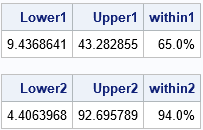I am not aware of a similar interpretation of the geometric coefficient of variation. The GCV is usually used to compare two samples. As opposed to the confidence intervals in the previous paragraph, the GCV does not make any reference to the geometric mean of the data.

### Other ways to compute the geometric mean

The methods in this article are the simplest ways to compute the geometric mean in SAS, but there are other ways.

• You can use the DATA step to log-transform the data, use PROC MEANS to compute the descriptive statistics of the log-transformed data, then use the DATA step to exponentiate the results.
• PROC SURVEYMEANS can compute the geometric mean (with confidence intervals) and the standard error of the geometric mean for survey responses. However, the variance of survey data is not the same as the variance of a random sample, so you should not use the standard error statistic unless you have survey data.

As I said earlier, there is some bad information out there on the internet about this topic, so beware. A site that seems to get all the formulas correct and present the information in a reasonable way is Alex Kritchevsky's blog.

You can download the complete SAS program that I used to compute the GM, GSD, and GCV. The program also shows how to compute confidence intervals for these quantities.

The post Compute the geometric mean, geometric standard deviation, and geometric CV in SAS appeared first on The DO Loop.